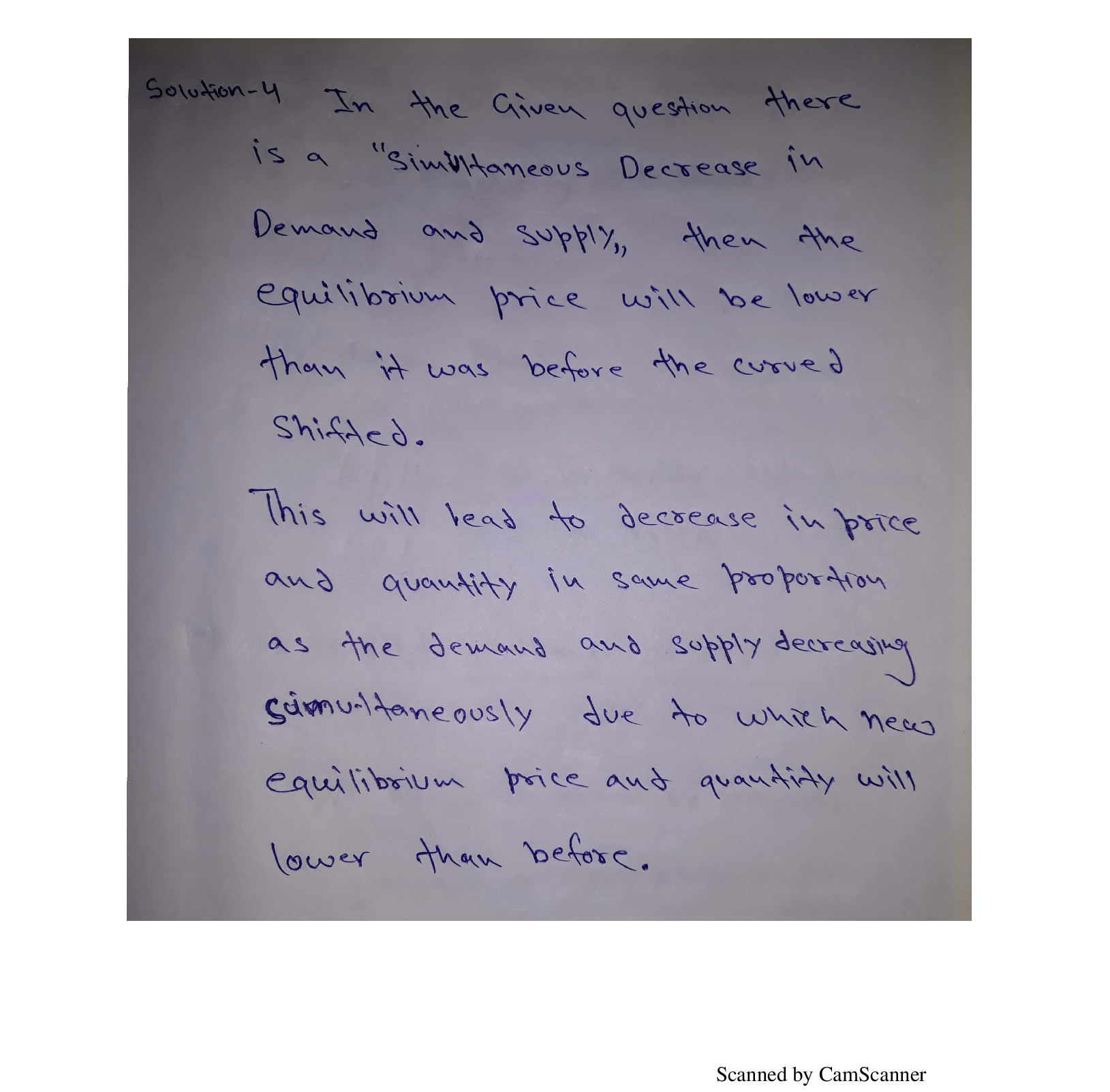Question

Microeconomics

4) Suppose a market starts in equilibrium; then we observe a decrease in supply and a decrease in demand simultaneously. What can we say about the new equilibrium? Illustrate.Verified### Question 39567Microeconomics

. A carpenter opens a firm producing bookcases. The inputs into the production process for bookcases are labour (L) and wood (K). In the short run, the carpenter can purchase wood at a price of £50/unit. The labour cost (the wage) is £10/hour.
a. Draw three is cost lines showing the different input combinations that yield total costs of £200, £500 and £1000.[10 marks]
b. The production function for bookcases is B(L,K) = min(2L, K). The carpenter is considering the input combinations given in the table below and has asked you to tell him how many bookcases he could produce in each case. Fill out the last column of the table, shaded in green, to indicate how many bookcases are produced in each case. Which of these input combinations, if any, is efficient, and why?
c. What are the Returns to Scale for the production function given in part b.?
d. The market for bookcases is competitive. Bookcases sell for £100 each. What is the profit or loss per bookcase produced in the short run? In the long run, would any new firms enter this market and what will happen to the price of bookcases?

### Question 31529Microeconomics

13) Suppose you have two different job offers. The first offer is in LA and pays \$8100 per month.The price of food (F) in LA is \$2 and the price of housing (H) is \$12. The second offer is in SanFrancisco and pays \$9600. Both food and housing are more expensive in SF at PF = 3 and PH = 14respectively. If your utility function is U (F, H) = FO.3 H 0.7 which job offer should you accept?

### Question 31528Microeconomics

12) In the market for smartphones, the price elasticity of supply is +0.8, and the price elasticity of demand is -1.2. At equilibrium, price is \$800 and quantity is 400000. (a) Assuming supply and demand are linear, reconstruct and draw the supply and demand curves. Label the intercepts. (b)To help consumers and phone-makers, the government proposes to subsidize smartphones by \$80each. What are PB and PS after the subsidy? What is the new equilibrium quantity? Illustrate them on the same graph. (c) Calculate the change in consumer surplus, producer surplus, government expenditure, and dead weight loss and identify them on the graph.

### Question 31527Microeconomics

11) A consumer's preferences for food (F) and clothing (C) are given by U(F,C) = F0.2C 0.8. The price of food is \$4, and the price of clothing is \$8. The consumer has an income of \$3600. a) What is the utility maximizing choice of food and clothing? b) How would the utility maximizing choice change if price of clothing increased to \$14? c) Given the answers to the previous parts plot a linear approximation to the demand function for clothing.

### Question 31526Microeconomics

10) If a consumer has chosen optimally, their Marginal Rate of Substitution is equal to the price ratio. Why is this? Interpret this in terms of the "price of utility" or "marginal utility per dollar" foreach good. PART TWO. Answer the following problems in the space provided. Please show your work in an organized way, with clearly labeled graphs if you use any. Questions are 10 points each.

### Question 31525Microeconomics

9) Draw a graph that shows the agricultural market in equilibrium with a binding price floor. Label equilibrium price, equilibrium quantity, and changes in consumer surplus, producer surplus, and deadweight loss on the graph. Is this price floor guaranteed to make producers better off?

### Question 31524Microeconomics

8) At the current market equilibrium, the price elasticity of demand for a certain good is much higher than the price elasticity of supply. If the government imposes a \$2 specific tax on this good,who will bear more of the burden the tax? Illustrate.

### Question 31523Microeconomics

7) Suppose that a consumer's marginal rate of substitution at her current chosen bundle is MUX /MUY = 4, but she is able to exchange X and Y at Px/PY = 3. Should she keep her current bundle,or can she make herself better off by trading at these prices? Which good will she buy, and which will she sell?

### Question 31522Microeconomics

6) The demand for a commodity is given by QD = 75 – 2P, and the supply by QS = 3 + P. What are the equilibrium price and quantity? If a tax of \$3 per unit is imposed, what will be the price and quantity after the tax?

### Question 31521Microeconomics

5) Good X becomes cheaper to produce, decreasing its price. As a result, the demand for good Y increases. What can you say about the cross-price elasticity between the two goods? Moreover,discuss whether the two goods are substitutes, complements or neither.

### Submit query